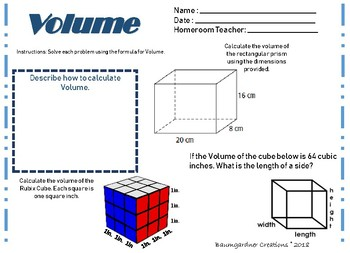# Perimeter, Area, and Volume Interactive Journal Pages and WorksheetsSubject
Resource Type
File Type

PDF

(750 KB|7 pages)
Standards
• Product Description
• Standards

Worksheets over Perimeter, Area, and Volume

Comes with Answer Key for each worksheet.

Use as a homework, check for understanding, and more.

Find the area of a rectangle with fractional side lengths by tiling it with unit squares of the appropriate unit fraction side lengths, and show that the area is the same as would be found by multiplying the side lengths. Multiply fractional side lengths to find areas of rectangles, and represent fraction products as rectangular areas.
Recognize volume as additive. Find volumes of solid figures composed of two non-overlapping right rectangular prisms by adding the volumes of the non-overlapping parts, applying this technique to solve real world problems.
Apply the formulas 𝘝 = 𝘭 × 𝘸 × 𝘩 and 𝘝 = 𝘣 × 𝘩 for rectangular prisms to find volumes of right rectangular prisms with whole-number edge lengths in the context of solving real world and mathematical problems.
Find the volume of a right rectangular prism with whole-number side lengths by packing it with unit cubes, and show that the volume is the same as would be found by multiplying the edge lengths, equivalently by multiplying the height by the area of the base. Represent threefold whole-number products as volumes, e.g., to represent the associative property of multiplication.
Relate volume to the operations of multiplication and addition and solve real world and mathematical problems involving volume.
Total Pages
7 pages
Included
Teaching Duration
N/A
Report this Resource to TpT
Reported resources will be reviewed by our team. Report this resource to let us know if this resource violates TpT’s content guidelines.